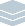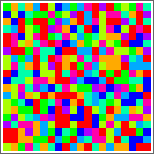Wolfram Archive
Wolfram Programming Lab is a legacy product.
All the same functionality and features, including access to Programming Lab Explorations, are available with Wolfram|One.
Start programming now. »
Try it now »
(no sign-in required)# Randomness of Pi

Test for randomness in the digits of π.

Run the code to find the numerical value of π to 50 digits. Try getting more than 50 digitsfor example, 1,000:

Pi represents the exact value of π . It cant be represented by a finite number of digits; thats why you dont see numerical digits when you evaluate it:

Pi

To turn Pi into a number, you have to specify how precise an approximation you want. This gives a 50-digit approximation to Pi:

N[Pi, 50]

N[Pi, 50]

Get a list of the first 100 digits of π in base 10. Try bases other than 10for example, 2:

This gives a list of the first 4 digits of π in base 10, followed by the number of digits to the left of the decimal point:

RealDigits[\[Pi], 10, 4]

Use First if you want just the list of digits:

First[RealDigits[\[Pi], 10, 4]]

First[RealDigits[Pi, 10, 100]]

Count how often 0 through 9 occur in the first 100 digits. Try more digits than 100:

Counts counts how often each element of a list occurs. This list has four ones and three twos:

Counts[{1, 2, 2, 2, 1, 1, 1}]

Counts[First[RealDigits[Pi, 10, 100]]]

Make a bar chart of 0 through 9 frequencies. See if it evens out with more than 100 digits:

Counts returns key-value pairs. In this case, the keys are the digits and the values are the number of times they occur:

Counts[First[RealDigits[Pi, 10, 100]]]

KeySort sorts by the keys, so you get the digit counts in order:

KeySort[Counts[First[RealDigits[Pi, 10, 100]]]]

This makes a chart of digit counts in digit order from 0 to 9. Mouse over a bar to see the numerical count:

BarChart[KeySort[Counts[First[RealDigits[Pi, 10, 100]]]]]

BarChart[KeySort[Counts[First[RealDigits[Pi, 10, 100]]]]]

Make a 20×20 array of π digits. Try sizes other than 20for example, 40:

Get the first 9 digits of π:

First[RealDigits[Pi, 10, 9]]

Partition the list into sublists of length 3:

Partition[First[RealDigits[Pi, 10, 9]], 3]

Format the list of lists as a grid. Each sublist is a row in the grid:

Grid[Partition[First[RealDigits[Pi, 10, 9]], 3]]

To make it easier to change the size of the grid, factor out the size using With. For an n×n grid of digits, you need n*n digits partitioned into lists of length n:

With[{n = 3}, Partition[First[RealDigits[Pi, 10, n*n]], n]]

Format that as a grid:

Grid[With[{n = 3}, Partition[First[RealDigits[Pi, 10, n*n]], n]]]

Now you can easily change the size of the grid from 3×3 to 4×4:

Grid[With[{n = 4}, Partition[First[RealDigits[Pi, 10, n*n]], n]]]

Grid[With[{n = 20}, Partition[First[RealDigits[Pi, 10, n*n]], n]]]

Visualize the array of digits. Try a larger array than 20×20:

Use ArrayPlot in place of Grid to show the digits graphically. Lower numbers are lighter and higher numbers are darker. Can you read off the digits {3,1,4,1,5,9,...} in the first row?

ArrayPlot[ With[{n = 20}, Partition[First[RealDigits[Pi, 10, n*n]], n]]]

The irregular, speckled appearance of the grid suggests that the digits of π are random. For comparison, here is a plot of the digits of 1/7, which are not random:

ArrayPlot[ With[{n = 20}, Partition[First[RealDigits[1/7, 10, n*n]], n]]]

ArrayPlot[ With[{n = 20}, Partition[First[RealDigits[Pi, 10, n*n]], n]]]

Specifying ColorFunctionHue makes a plot with colors instead of gray values. It shows the randomness of the digits of π, but its not as easy to make the correspondence between colors and digits as it is between gray values and digits:

ArrayPlot[ With[{n = 20}, Partition[First[RealDigits[Pi, 10, n*n]], n]], ColorFunction -> Hue]

ArrayPlot[ With[{n = 20}, Partition[First[RealDigits[Pi, 10, n*n]], n]], ColorFunction -> Hue]

Make a π random walk. Try it for more than 100 digits:

This gives the first 100 digits of π in base 2:

First[RealDigits[Pi, 2, 100]]

Subtracting .5 from each digit gives -.5 for a 0 and .5 for a 1:

First[RealDigits[Pi, 2, 100]] -.5

Accumulate gives successive sums of the items in the list, namely {.5,.5+.5,.5+.5-.5,...}:

Accumulate[First[RealDigits[Pi, 2, 100]] - .5]

If the digits of π are truly random, youd expect there to be about as many 0s as 1s, which means that the accumulated sums should hover around zero.

Plot the sums to see if thats the case:

ListLinePlot[Accumulate[First[RealDigits[Pi, 2, 100]] - .5]]

They seem to be drifting away from 0. Does that trend continue? Have a look at more digits (1,000 this time):

ListLinePlot[Accumulate[First[RealDigits[Pi, 2, 1000]] - .5]]

You can do the same analysis in other bases. Just remember to subtract the average of the digits up to the base 1. For base 3 digits, you need to subtract (0+1+2)/3=1 (if the base is b, the average of the base b digits is (b-1)/2):

ListLinePlot[Accumulate[First[RealDigits[Pi, 3, 100]] - 1]]

ListLinePlot[Accumulate[First[RealDigits[Pi, 2, 100]] - .5]]